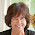## Monday, February 06, 2017

### Monday Poetry Stretch - Rhupunt

I am still reading and pondering the forms in Robin Skelton's The Shapes of Our Singing: A Comprehensive Guide to Verse Forms and Metres from Around the World. The Rhupunt is a Welsh verse form. Lines are 4 syllables long, with the last line rhyming with the last line of the following stanza. Stanzas may be 3, 4, or 5 lines long. Here is the pattern for these versions.

3-line

x x x A
x x x A
x x x B

x x x C
x x x C
x x x B

4-line
x x x A
x x x A
x x x A
x x x B

x x x C
x x x C
x x x C
x x x B

5-line
x x x A
x x x A
x x x A
x x x A
x x x B

x x x A
x x x A
x x x A
x x x A
x x x B

Since the lines in each stanza are generally thought to be portions of a long line, they are sometimes presented as a couplet with lines of 12 to 20 syllables. Written this way the rhupunt would look like this:
x x x A x x x A x x x A x x x B
x x x C x x x C x x x C x x x B

You can read more about the rhupunt at The Poets Garret.

I hope you'll join me this week in writing a rhupunt. Please share a link to your poem or the poem itself in the comments.

#### 1 comment:

1.There once was a president Trumpet
Whose wife some considered a strumpet.
He screeched and he blared
Till no voter still cared,
And the senate got brave and then dumped it.